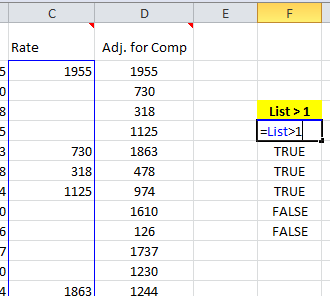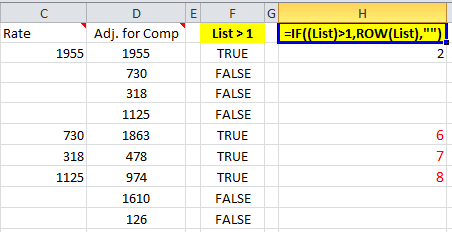# Formula Forensic 014 – Faseeh’s Formula

In early February Subhapratimdas asked a question at the Chandoo.org Forums.

I have a large list which includes blanks, I want to retrieve the list without the blanks

Faseeh, responded with a neat array formula

=IFERROR(OFFSET(\$C\$2,SMALL(IF((List)>1,ROW(List),””),ROW(E1))-2,0),”…”)

Old Chippy thought it was neat and nominated it for a Forensic examination.

So today we will pull Faseeh’s Formula apart to see what’s inside.

## Faseeh’s Formula

As usual we will work through this formula using a sample file for you to follow along. Download Here.

Faseeh’s formula is an Array Formula:

=IFERROR(OFFSET(\$C\$2,SMALL(IF((List)>1,ROW(List),””),ROW(E1))-2,0),”…”)

This is the formula taken from Cell D2. This is important but we will come back to it later.

A quick look shows that it is an Offset Formula surrounded in an IFERROR wrapper

=IFERROR(OFFSET(\$C\$2,SMALL(IF((List)>1,ROW(List),””),ROW(E1))-2,0),”…”)

The IFERROR() function is a new function introduced in Excel 2007. Its use it to look at its first component and return its value, but if the first component returns an error then return the value in the second component, in this case “…”

So the real formula is

OFFSET(\$C\$2,SMALL(IF((List)>1,ROW(List),””),ROW(E1))-2,0)

And if this returns an error, then IFERROR will kick in and return a “…” instead of an error.

Lets look at the main part

OFFSET(\$C\$2,SMALL(IF((List)>1,ROW(List),””),ROW(E1))-2,0)

The OFFSET function has the syntax

OFFSET(reference, rows, cols, [height], [width])

In our example

OFFSET(\$C\$2,SMALL(IF((List)>1,ROW(List),””),ROW(E1))-2,0)

So the Offset Formula is using

Reference: \$C\$2

Rows: SMALL(IF((List)>1,ROW(List),””),ROW(E1))-2

Columns: 0

So Offset is looking at a Reference of C2 and then looking down SMALL(IF((List)>1, ROW(List), “”), ROW(E1))-2 rows and across 0 cells to return a new reference.

So what is SMALL(IF((List)>1,ROW(List),””),ROW(E1))-2 doing.

Next thing to notice is that there is a Named Formula “List” used twice in the formula

Looking at the name manager (Keyboard ShortcutCtrl F3), we can see that List is a straight range reference to C2:C10000

List : =Sheet1!\$C\$2:\$C\$10000

Back to

SMALL(IF((List)>1,ROW(List),””),ROW(E1))-2

If we highlight the components

SMALL(IF((List)>1,ROW(List),””),ROW(E1))-2

We can now see that the formula is using the SMALL() function

The syntax of the SMALL function is:

SMALL(array, k)

So this shows that the SMALL(IF((List)>1,ROW(List),””),ROW(E1))-2

Will get the item number Row(E1) from the array IF((List)>1,ROW(List),””) and then subtract 2 from it.

The Row(E1) is important as you will remember way back at the start I mentioned that this is the formula from D2, so Row(E1) is getting the Row number 1 cell above the current Row.

This means that in Row 2, it is getting the 1st item (Row 1) from the Small function, in Row 3 it is getting the 2nd and in row 10 it is getting the 9th item from the Small function, etc.

So what array is Small() looking up?

IF((List)>1,ROW(List),””)

This is a very neat and quick method of setting up an array

The Named Formula “List” is an Array which has received the values from Sheet1!\$C\$2:\$C\$10000, so it is a 9999 rows by 1 column array

A quick way to check this is to enter in F5:F10, =List>1 and press Ctrl EnterWhen the corresponding row has a Value > 1, =List>1 returns TRUE

When the corresponding row has a Blank cell, =List>1 returns FALSE

Stepping out

=IF((List)>1,ROW(List),””)

If we step out to the If formula we see that if ( the value in the Array >1, put the Row(List) else “”So in H2:H10 array enter =IF((List)>1,ROW(List),””) Ctrl Shift Enter

You can see that this is creating an Array of the Row Numbers that aren’t blank

Back to our SMALL() function

SMALL(IF((List)>1, ROW(List), “”), ROW(E1))-2

This is now reading like, return the smallest Row(Current Row -1) from the array of items which has removed the blanksSo If we step out again, and look at 3 cells: D2:D4

D2 will return the 1st smallest Number from the array Row(E1), which is a Value of 2

D3 will return the 2nd smallest Number from the array Row(E2), which is a Value of 6

D4 will return the 3rd smallest Number from the array Row(E2), which is a Value of 7

But these values are the Row Offset in the original Offset function

OFFSET(\$C\$2, SMALL(IF((List)>1,ROW(List),””), ROW(E1))-2, 0)

So in:

D3: OFFSET(\$C\$2, SMALL(IF((List)>1,ROW(List),””), ROW(E1))-2, 0)

= OFFSET(\$C\$2, 2-2, 0)

= C2

= 1955

D4: OFFSET(\$C\$2, SMALL(IF((List)>1,ROW(List),””), ROW(E1))-2, 0)

= OFFSET(\$C\$2, 6-2, 0)

= OFFSET(\$C\$2, 4, 0)

= C6

=730

D5:      OFFSET(\$C\$2, SMALL(IF((List)>1,ROW(List),””), ROW(E1))-2, 0)

= OFFSET(\$C\$2, 7-2, 0)

= OFFSET(\$C\$2, 5, 0)

= C7

= 318

Once the formula tries to retrieve values past the end of the data the formula returns an error and the IFERROR() function returns a “…”

## Formula Forensics “The Series”

Formula Forensic Series

I have received a few more ideas since last week and these will feature in coming weeks.

I do need more ideas though and so I need your help.

If you have a neat formula that you would like to share and explain, try putting pen to paper and draft up a Post like above or;

If you have a formula that you would like explained but don’t want to write a post also send it to Chandoo or Hui.Hello Awesome...

My name is Chandoo. Thanks for dropping by. My mission is to make you awesome in Excel & your work. I live in Wellington, New Zealand. When I am not F9ing my formulas, I cycle, cook or play lego with my kids. Know more about me.

Thank you and see you around.

### Related articles:

 Written by Hui... Tags: Formula Forensics, if(), iferror, OFFSET(), row(), small Home: Chandoo.org Main Page ? Doubt: Ask an Excel Question

### 12 Responses to “Formula Forensic 014 – Faseeh’s Formula”

1.Eric says:

Very Cool!

And you can use the SMALL function to then sort the results.

=IFERROR(SMALL(OFFSET(List,0,1),ROW(E2)),"...")

Use as an array function, and copy down.

2.Eric says:

Oops... forgot the ROW(E2)-1

Here's the correction:

=IFERROR(SMALL(OFFSET(List,0,1),ROW(E2)-1),”…”)

3.SirJB7 says:

Hi, Faseeh!
Congratulations for deserving a Formula Forensic article.
My best regards!

4.Faseeh says:

@SirJB7,

Thank You Sir! 🙂
....and thanks to Hui & Oldchippy for explaining & referring it!!

Yours truly
Faseeh

5.Jova says:

What I usually do is I add another row where I put sequential numbers from 1 to n (even for the blanks).
Select both rows and sort descending by the data row.
Select both rows again, but just where the data is, and sort ascending by sequential number.

6.Fred says:

Very neat!

Just one question, why wouldn't "Skip Blank" (under paste special) work? I tried copying the data with blank data, paste special as skip blank but it didn't work.

I have seen this "Skip Blank" and I thought this would be the place to go but never have a real life chance of using it.

Could someone shed some light on this please? Thanks.

7.Eric says:

Well, Skip Blank might work, but the cool thing about the formula is that, as you add data to your table (and if your table is set up correctly to expand with new rows) Faseeh's formula is added in automatically and the blanks are automatically put to the bottom of the list. No need to do any key strokes and repaste in the column of data! Same thing with my use of SMALL() to sort the column.

This is real useful in dashboards. You don't want to have to go in every time you add data and recreate the dashboard by doing a whole bunch of keystrokes.

8.Sajan says:

Great formula!

And here is the same formula tweaked to avoid the volatile OFFSET function:
=IFERROR(INDEX(A:A,SMALL(IF((list)>0,ROW(list)),ROW(B1))),"…")

entered using Ctrl+Shift+Enter

(The formula assumes that the list with blanks is in column A, and that this formula is put in column B, starting in cell B1.)

Cheers,
Sajan.

9.Kittu20 says:

Hi,

Its very nice way of explaining and thanks Chandoo and Faseeh.
I have a query. When I'm using IF(List>1, ROW(List),"") , I am always getting 2 as output. Can you help me where I must be going wrong?

THanks
Krishna

10.Aleks Z says:

Thanks Faseeh,

I'm using this formula to filter out gaps in a set of data so that I can use the "RSQ" and "LINEST" functions for non-linear trendline coefficients.

I have managed to use your example to condense the data and remove all the gaps. Any suggestions how to set up a formula that recognizes the "end" of the dataset (i.e, where the data stops)?

For example, how could I modify the general formula below to determine the RSQ for a logarithmic trend of a set of data which I have just condensed with the "Faseeh" formula?

RSQ (logarithmic trend) = RSQ(Y,LN(X))

Aleks

•Hui... says:

@Aleks
Normally a formula like:
Xvalues: =Offset(A1,,,counta(a:a),1)
can be used to setup a range of all the data
same for Y
=Offset(B1,,,counta(b:b),1)
or you can offset from the first

=Offset(Xvalues,,1)

11.Philippe says:

Great formula !! Thanks for it and these clear explanations

But I have a small problem with it: if a cell in column D has a value of 1 then it is considered as blank; if you use List>=1 then 0 is considered as blank... Am I wrong somewhere?

 « 14 ways to check if an year is leap year, using Excel [just for fun] Do you use Pivot Tables? What do you use them for & where do you struggle [Survey] »

### Get FREE Excel & Power-BI Newsletter

One email per week with Excel and Power BI goodness. Join 100,000+ others and get it free.